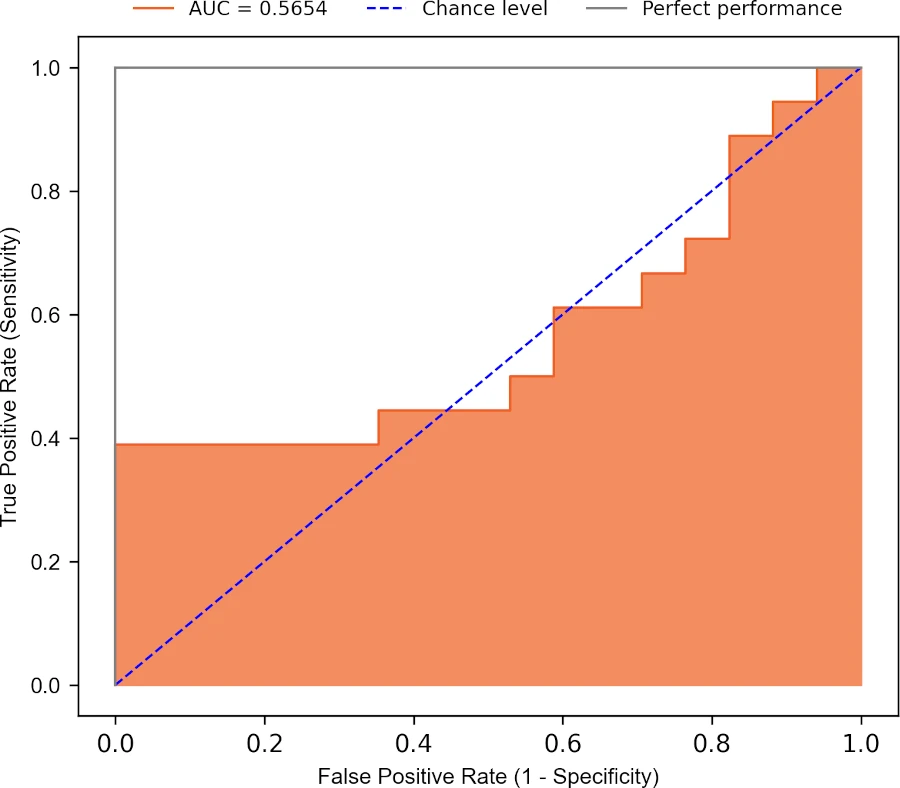# How to Plot ROC Curve in Python

The Receiver Operating Characteristic (ROC) curve is a graphical plot for evaluating the performance of binary classification models such as logistic regression, support vector machines, etc.

ROC curve visualizes the trade-off between sensitivity (true positive rate) and specificity (false positive rate) for all possible threshold values.

A model with good predictability will have ROC curve that extends towards the upper-left corner of the plot (high true positive rate and low false positive rate). A perfect prediction model will have an ROC curve with `true positive rate (TPR)` = 1 and `false positive rate (FPR)` = 0.

In addition, the ROC curve summarises the model predictability based on the area under the ROC curve (AUC). AUC ranges from 0 to 1, and a model with higher a AUC (close to 1) has higher predictability.

In Python, the ROC curve can be plotted using the `roc()` function from the `bioinfokit` package.

We will take the example of the logistic regression to plot the ROC curve in Python.

## Getting the dataset

We will use the sample breast cancer dataset for fitting the logistic regression model.

This sample breast cancer dataset includes four features (predictors) and outcome [patient is healthy (0) or cancerous (1)].

``````# import package
import pandas as pd

# view first few rows
# Classification is the outcome with two levels with cancer (1) or healthy (0) patients
Age        BMI  Insulin  Leptin  Classification
0   48  23.500000    2.707  8.8071               0
1   83  20.690495    3.115  8.8438               0
``````

## Train-Test split

Split the dataset into train and test datasets. We will use the `train_test_split()` function from the `sklearn` package to split 70% as training and 30% as test datasets.

The training dataset will be used for training the model and the test dataset will be used for prediction.

``````# import package
from sklearn.model_selection import train_test_split

# split into training and testing
df_train, df_test = train_test_split(df, train_size = 0.7, random_state = 0)
``````

## Fit the logistic regression model and perform prediction

Fit the logistic regression model using training dataset,

``````# import package
from sklearn.linear_model import LogisticRegression

# get X and y
X_train = df_train[["Age", "BMI", "Insulin", "Leptin"]]
y_train = df_train["Classification"]

# fit the model
fit = LogisticRegression(random_state = 0).fit(X_train, y_train)

# perform prediction
# get X and y
X_test = df_test[["Age", "BMI", "Insulin", "Leptin"]]
y_test = df_test["Classification"]

# calculate predicted probabilities
pred_probs = fit.predict_proba(X_test)[:, 1]
``````

## Plot ROC curve

We will use the `roc()` function from the `bioinfokit` to plot the ROC curve. ROC plot requires TPR (sensitivity) and FPR (specificity) values.

Calculate TPR and FPR for ROC,

``````# import package
from sklearn.metrics import roc_curve, roc_auc_score

# calculate FPR and TPR and AUC
fpr, tpr, thresholds = roc_curve(y_true = y_test, y_score = pred_probs)
auc = roc_auc_score(y_true = y_test, y_score = pred_probs)
``````

Now, plot the ROC curve,

``````# import package
from bioinfokit.visuz import stat

# plot ROC
stat.roc(fpr = fpr, tpr = tpr, auc = auc, shade_auc = True, per_class = True,
legendpos='upper center', legendanchor=(0.5, 1.08), legendcols=3)
``````Based on the ROC curve and AUC (0.56), the model has poor predictability. The model will not perform well in classifying the healthy and cancer patients.

Related: Calculate AUC in Python

## Subscribe to get new article to your email when published

* indicates required